Courses

# Principle of Virtual Work for a Particle and a Rigid body Mechanical Engineering Notes | EduRev

## Mechanical Engineering : Principle of Virtual Work for a Particle and a Rigid body Mechanical Engineering Notes | EduRev

The document Principle of Virtual Work for a Particle and a Rigid body Mechanical Engineering Notes | EduRev is a part of the Mechanical Engineering Course Engineering Mechanics - Notes, Videos, MCQs & PPTs.
All you need of Mechanical Engineering at this link: Mechanical Engineering

Principle of Virtual Work

Degrees of Freedom Associated with the concept of the lumped-mass approximation is the idea of the NUMBER OF DEGREES OF FREEDOM. This can be defined as “the number of independent co-ordinates required to specify the configuration of the system”. The word “independent” here implies that there is no fixed relationship between the coordinates, arising from geometric constraints.

Degrees of Freedom of Special Systems

A particle in free motion in space has 3 degrees of freedom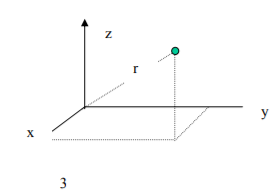particle in free motion in space has 3 degrees of freedom

If we introduce one constraint – e.g. r is fixed then the number of degrees of freedom reduces to 2. note generally: no. of degrees of freedom = no. of co-ordinates –no. of equations of constraint

Rigid Body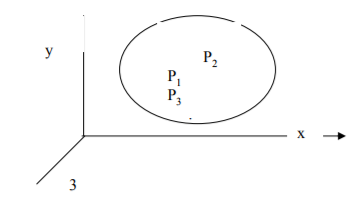This has 6 degrees of freedom 3 translation 3 rotation

e.g. for partials P1, P2 and P3 we have 3 x 3 = 9 co-ordinates but the distances between these particles are fixed – for a rigid body – thus there are 3 equations of constraint.

The no. of degrees of freedom = no. of co-ordinates (9) - no. of equations of constraint (3) = 6.

Formulation of the Equations of Motion

Two basic approaches:

1. application of Newton’s laws of motion to free-body diagrams Disadvantages of Newton’s law approach are that we need to deal with vector quantities – force and displacement. thus we need to resolve in two or three dimensions – choice of method of resolution needs to be made. Also need to introduce all internal forces on free-body diagrams – these usually disappear when the final equation of motion is found.
2. use of work with work based approach we deal with scalar quantities – e.g. work – we can develop a routine method – no need to take arbitrary decisions.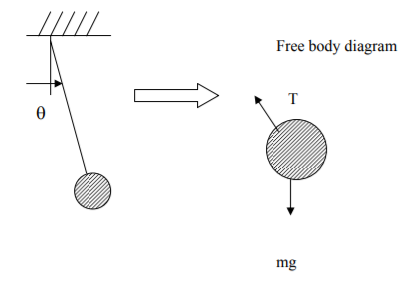Principle of Virtual Work

The work done by all the forces acting on a system, during a small virtual displacement is ZERO.

Definition A virtual displacement is a small displacement of the system which is compatible with the geometric constraints.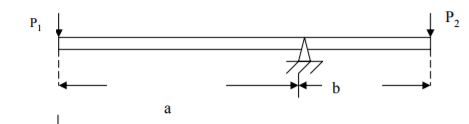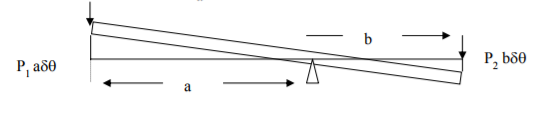e.g. This is a one-degree of freedom system, only possible movement is a rotation.

work done by P1 = P1(- aδθ)

work done by P2 = P2(bδθ)

Total work done = P1(- aδθ) + P2(bδθ) = δW

By principle of Virtual Work

δW = 0

therefore:

P1 (- aδθ) + P2(bδθ) = 0

- a P1 + bP2 = 0

P1a = P2b

D’Alembert’s Principle

Consider a rigid mass, M, with force FA applied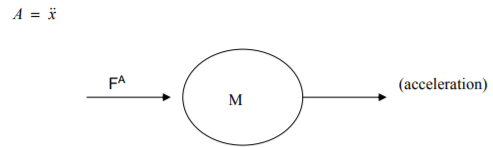From Newton’s 2nd law of motion

FA= Ma =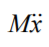or

FA== 0

Now, the term ( −) can be regarded as a force – we call it an inertial force, and denote it FI – thus

we can then write: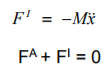In words – the sum of all forces acting on a body (including the inertial force) is zero – this is a statics principle. In fact all statics principles apply if we include inertial forces, including the Principle of Virtual Work.

Offer running on EduRev: Apply code STAYHOME200 to get INR 200 off on our premium plan EduRev Infinity!

,

,

,

,

,

,

,

,

,

,

,

,

,

,

,

,

,

,

,

,

,

;